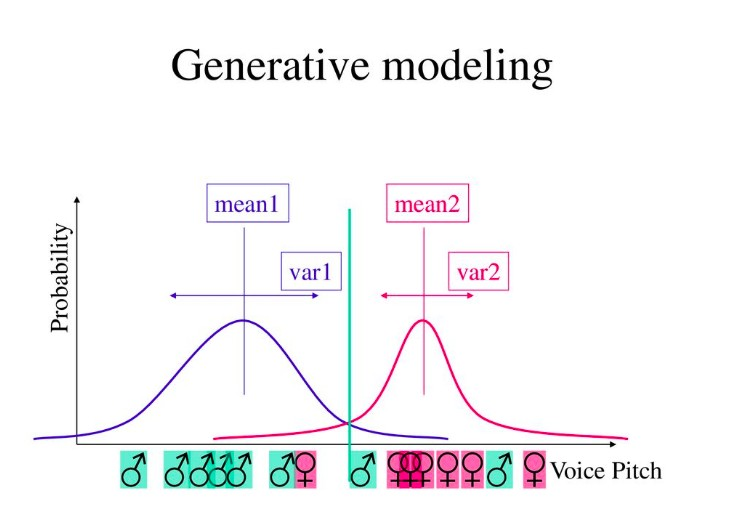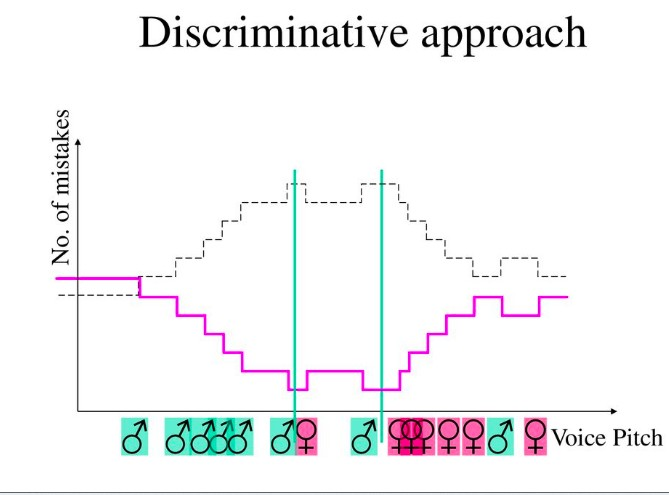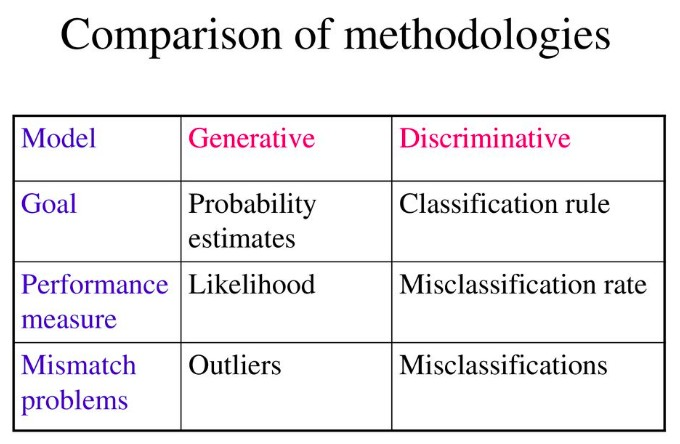# Generative vs Discriminative ModelGenerative Model and Discriminative model are different way to categorize the learning algorithm.Both Generative and Discriminative Model can be used for classification and both can be supervised.But the way they go about seperating the positive and neagitive class are different.

## Generative Model

• In Generative approachs, typically what it try to do, it build a model of positive and model of negative(what I meant by the model is that it is some characterisation of the entire population of positives) and try to come with some way to characterize a distribution of what kind of positive are you likely to see and what kind of positive you not likely to see and it will do same thing for negative.

• Then if you ask a generative model to do classification, what it will do, It will look for a boundary in space where one model become more plausible or more likely than another model.

• Suppose you have features like positive and negative, It will Compute the probability and when the probability crosses a particular boundary that's where It thinks about decision boundary.

• Few examples of Generative Classifier are :

• Naive Bayes.
• Bayesian networks
• Markov random fields
• Hidden Markov Models (HMM)
• In more formal way :- Generative classifiers learn a model of the joint probability, p( x, y), of the inputs x and the label y, and make their predictions by using Bayes rules to calculate p(ylx), and then picking the most likely label y.

## Let's try to figure it out using Voice Pitch Examples

Let's suppose we have a model in which we have to identify the voice of male and female. Only "Pitch of the voice" is given as a single paramenter.Intutively we have to find a threshold pitch such that if it below that threshold we would say male and above that we would say female.## How to find the thresold Pitch ?

One way of doing is to use generative modelling,it will try to plot probabilistic distribution for male and female( Here distribution refers to normal distribution).It will find the threshold pitch where the two distribution intersect.

What this threshold states - If ptich is above that threshold, It's more likely to female and If it is below that threshold, It's more likely to male.
Key points abouts Generative Model --- It produces random sample from the distribution that are observed.

## Discriminative Model

• On the other hand, Discriminative model are more powerful model than Generative Model.In simple word what is does, It puts all of its effort and work into modelling the boundary between two classes. The boundary is not necessary going to be linear, It can take any form.

• It doesn't care about the data point out there that are away from the boundary(outliers points), what it cares about, is there any line that seperates the positive from the negatives.

• It really looks for the points that are closest to the boundary to make the decision. An Disciminative approach can ignore most of your data even labeled data.it will just focus on the important one.

• Discriminative approach is more powerfull when you have lots of training examples.But you cann't use it on unlabbeled data or unsupervised tasks.

• In more formal ways : Discriminative classifiers model the posterior p(ylx) directly, or learn a direct map from inputs x to the class labels

• Few examples of Discriminative Classifier are :

• Logistic regression
• Scalar Vector Machine
• Nearest neighbour
• Conditional Random Fields (CRF)s

## Let's try to figure it out using Voice Pitch Examples

Using the same Voice Pitch Model, But now we will be using Discrimiative approach to identify the male and female voice.It's approach is very different from the generative approach.It basically says, I don't care about the what the distribution is. I just cares about the error in my threshold.

It looks for all possible threshold as they were passing through and decides where it have smallest no of mistakes and It tries all possible plot.

It draws different graph. It plot the graph using "No of Mistakes vs Threshold Pitch". In above graph, the lower portion states the no of mistakes for threshold where female pitches higher than males and upper black portion states that no of mistakes for threshold where male pitches higher than females.

Clearly the upper portion in case of no of mistakes is much worse.So we can choose the threshold from lower portion which is best. In above case, two value of threshold are optimum.You can choose any one of them.

## Summary